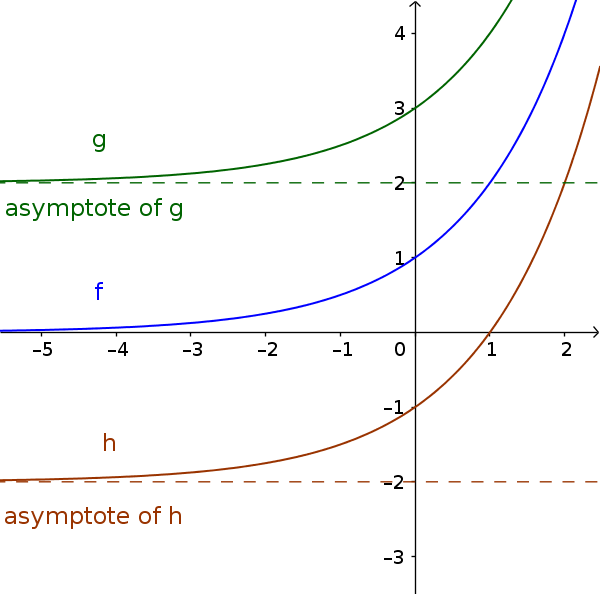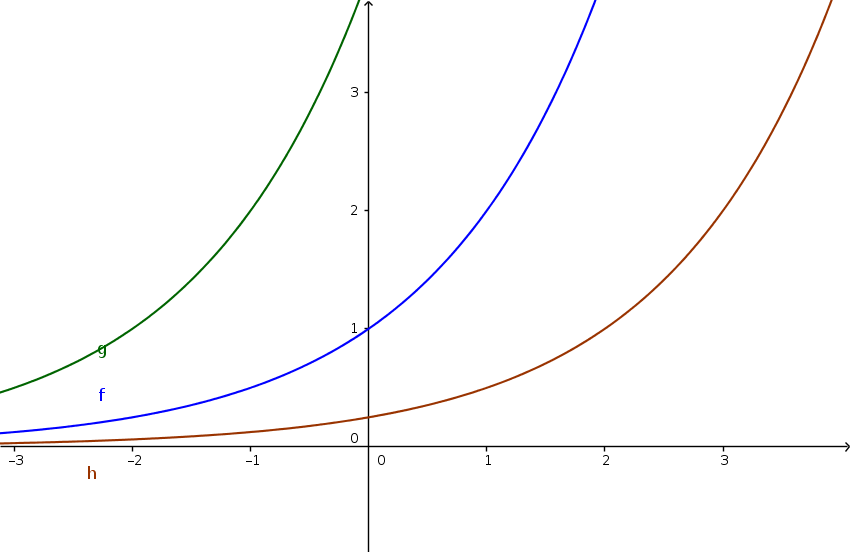Math Exponential functions Shifting

# Shifting

## a) along the y-axis

The graph of an exponential function is shifted with the parameter $d$ along the y-axis. This changes the asymptote and the value range. The general formula is:

$y=b^x+d$
!

### Remember

• If $d > 0$, the graph is shifted up.
• If $d < 0$, the graph is shifted down and gets a zero.

• The asymptote is at $y=d$.
• The range of values is $W=[d,\infty]$

### Example

$\color{blue}{f(x)=2^x}$
$\color{green}{g(x)=2^x+2}$
$\color{brown}{h(x)=2^x-2}$## b) along the x-axis

The graph of an exponential function is shifted with the parameter $c$ along the x-axis. The general formula is:

$y=b^{x+c}$
!

### Remember

• If $c$ > 0, the graph is shifted to the left and corresponds to a stretch of $b^c$.
• If $c$ < 0, the graph is shifted to the right and corresponds to a compression of $(\frac{1}{b})^c$.

### Beispiel

$\color{blue}{f(x)=2^x}$
$\color{green}{g(x)=2^{x+2}}$
$\color{brown}{h(x)=2^{x-2}}$